## 哈希表

### 哈希表的一些基本概念

1. 哈希表（Hash Table）：也叫散列表，是根据关键码值（Key-Value）而直接进行访问的数据结构，也就是我们常用到的map。

2. 哈希函数：也称散列函数，是Hash表的映射函数，它可以把查找表中的关键字映射成该关键字对应的地址函数，表示如下：
$$Hash(key) = Addr,（地址可以是数组下标、索引、内存地址等）$$
哈希函数能使对一个数据序列的访问过程变得更加迅速有效，通过哈希函数数据元素能够被很快的进行定位。

3. 冲突：两个不同的关键字，由于散列函数值相同，因而被映射到同一表位置上。该现象称为冲突(Collision)或碰撞
• 尽量减少冲突
• 冲突不可避免，要设计好处理冲突的方法

### 散列函数的构造方法

1. 定义域必须包含全部需要存储的关键字
2. 地址等概率，均匀的分布在整个地址空间
3. 函数尽量简单，较短时间内能算出任一关键字地址

### 哈希表的定址方法

1. 直接定址法：直接取关键字的某个线性函数值作为散列地址，例如：
$$H(key) = a \times key + b,(a和b均为常数)$$

1. 除留余数法：(最常用) 假定表长为m，取一个不大于m但最接近或者等于m的质数P，例如：
$$H(key) = key\%P$$
2. 数字分析法：比如有一组$value1=112233，value2=112633，value3=119033$，针对这样的数我们分析数中间两个数比较波动，其他数不变。那么我们取key的值就可以是$key1=22,key2=26,key3=90$。

1. 平方取中法：取关键字的平法值的中间几位作为散列地址。

1. 折叠法：举个例子，比如$value=135790$，要求key是2位数的散列值。那么我们将$value$变为$13+57+90=160$，然后去掉高位$“1”$,此时$key=60$，这就是他们的哈希关系。这样做的目的就是key与每一位value都相关，来达到“散列地址”尽可能分散的目的。

### 哈希表解决冲突的方法

1. 开放定址法：
如果两个数据元素的哈希值相同，则在哈希表中为后插入的数据元素另外选择一个表项。当程序查找哈希表时，如果没有在第一个对应的哈希表项中找到符合查找要求的数据元素，程序就会继续往后查找，直到找到一个符合查找要求的数据元素，或者遇到一个空的表项。

$$H_{i} = (H(key)+d_{i})\%m$$

• 线性探测法：$d_i=1,2,…, m-1$，可能造成大量元素在相邻地址聚集，降低查找效率。
• 平方探测法：$d_i=1^2,-1^2,2^2,-2^2,…,-k^2,(k<=m/2)$，m必须是一个可表示为$4k+3$的质数。避免出现“堆积问题”，但是不能探测全部单元（至少一半）。
• 再散列法：$d_i=Hash_{2}(key)$，使用2个散列函数。
• 伪随机序列法：$d_i=$伪随机序列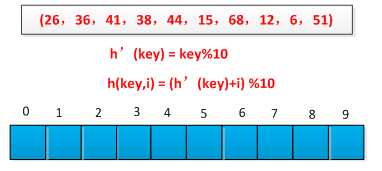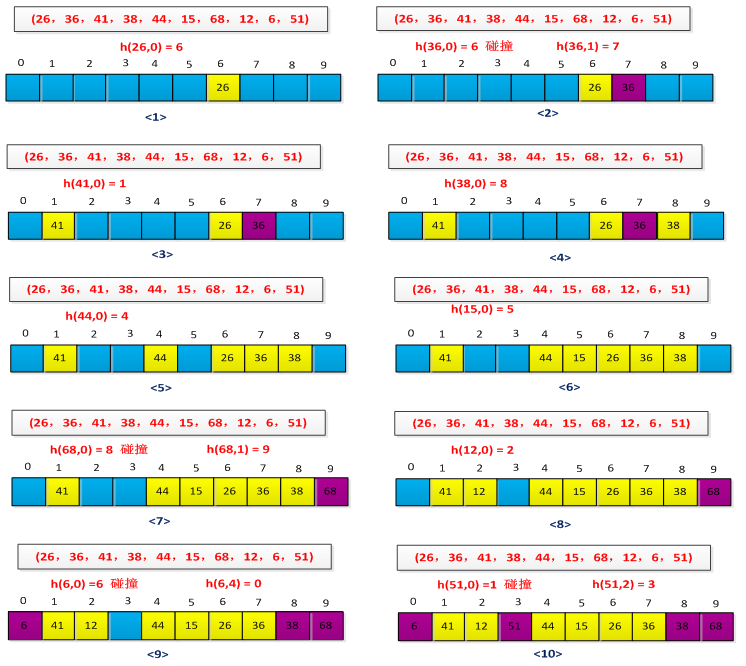1. 拉链法：
将哈希值相同的数据元素存放在一个链表中，在查找哈希表的过程中，当查找到这个链表时，必须采用线性查找方法。适用于经常进行插入、删除的情况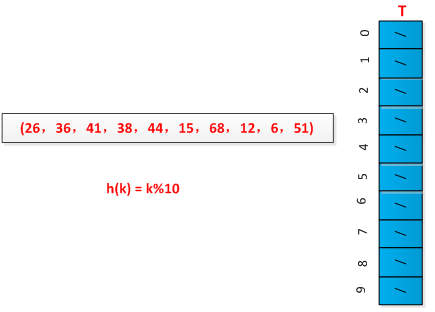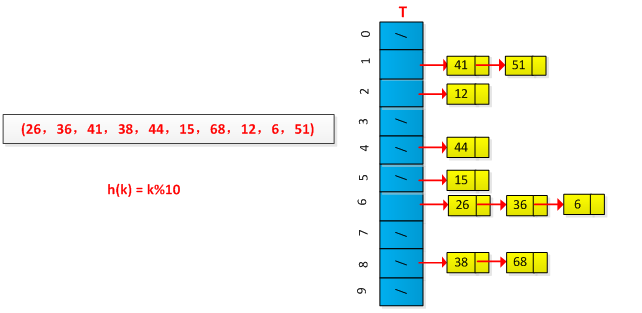### 散列查找及性能分析

$$\alpha = \frac{表中记录数}{散列表长度} = \frac{n}{m}$$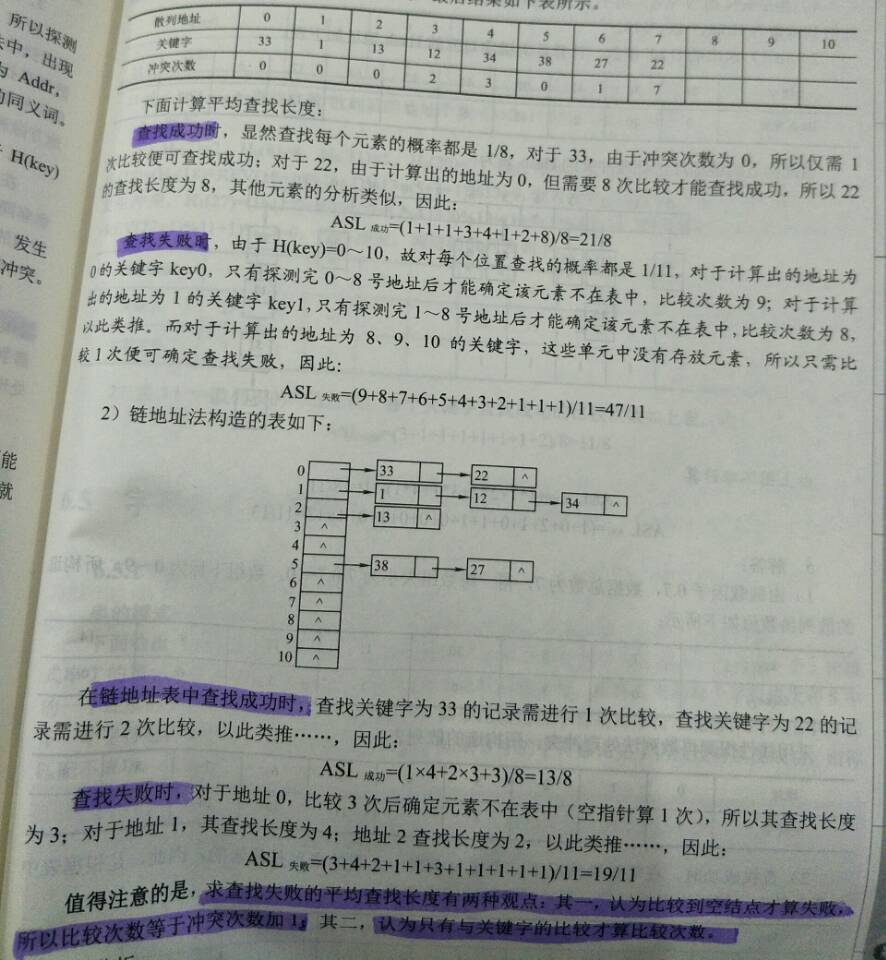1. 将字符串的所有的字符的ASCII码值进行相加，将所得和作为元素的关键字
2. 假设关键字至少有三个字母构成，散列函数只是取前三个字母进行散列
3. 借助Horner’s 规则，构造一个质数（通常是37）的多项式，（非常的巧妙，不知道为何是37）。计算公式为:$key[keysize-i-1]*37^i, 0<=i<keysize$求和。

## 哈希查找

1. 用给定的哈希函数构造哈希表；
2. 根据选择的冲突处理方法解决地址冲突；
3. 在哈希表的基础上执行哈希查找。

Hash那点事儿

------ 本文结束------